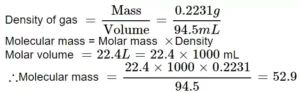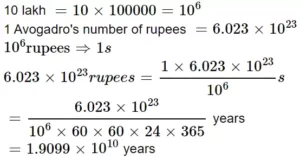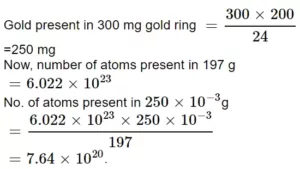# Important Solved Questions on Mole ConceptImportant Solved Questions on Mole Concept

Que 1. Calculate the number of atoms of each type in 5.3 gram of sodium carbonate (Na2CO3).\
Ans 1. Given mass of sodium carbonate (Na2CO3) = 5.3 g
​Molar mass of sodium carbonate = 23 × 2 + 12 + 16 × 3 = 106g/mol
Number of moles = given mass/ Molar mass = 5.3/106 =   0.05 mol
Now, 1 mole of Na2CO3 contains = 2 × 6.022 × 1023 sodium atoms
∴0.05 of Na2CO3 contains = 0.05 × 2 × 6.022 × 1023 sodium atoms
= 6.022 × 1022 = 6.022 × 1022 Na atoms.
1 mol of Na2CO3 contains  = 6.022 × 1023 carbon atoms
0.05 mol of Na2CO3 contains  = 0.05 × 6.022 × 1023 carbon atom
∴0.05 of Na2CO3 contains  = 0.05 × 3 × 6.022 × 1023 oxygen atoms
= 9.03 × 1023 = 9.03 × 1023 oxygen atoms.

Que 2. Chlorophyll, the green colouring matter of plants responsible for photosynthesis, contains 2.68% of magnesium by mass. Calculate the number of magnesium atoms in 2.00 g of chlorophyll.
Ans 2. Mass of chlorophyll = 2.0 g
Percentage of Mg = 2.68 g
Mass of Mg in 2.0 g of chlorophyll = 2.68 × 2.0/100 = 0.054 g
6.022 × 1023 atoms of magnesium = 24 g
24 g of Mg contains = 6.022 × 1023 atom
0.054 g of Mg contains = 6.022 × 12.0/24 = 1.3 × 1021 atoms

Que 3. The mass of 94.5 mL of a gas at S.T.P. is found to be 0.2231 g. Calculate its molecular mass.
Ans 3.Important Solved Questions on Mole Concept

Que 4. Calculate the number of molecules and number of atoms present in 11.2 liters of oxygen (O2) at N.T.P.
Ans 4. 1 mole occupies 22.4 litres at STP
Thus 11.2 litres contain = 11.2/22.4 = 0.5 moles
1 mole contains 6.03 ×1023 = 3.0115 × 1023 molecules
We know 1 molecule contains 2 atoms
3.0115 × 1023 molecules contain = 2 × 3.0115 × 1023 = 6.023 × 1023 atoms.

Que 5. Calculate the weight of carbon of monoxide having the same number of oxygen atoms as are present in 88 g of carbon dioxide.
Ans 5. Molecular mass of CO2 = 12 + 2 × 16 = 44
1mol of CO2 = 44 g
44 g of CO2 contain = 6.022 × 1023 molecules
88 g of CO2 contain = 6.022 × 1023 × 88/44 = 12.04 × 1023 molecules
Each molecule of CO2 contain 2 oxygen atoms
No. of oxygen atoms in 12.04 × 1023 molecules of CO2 = 2 × 12.04 × 1023 = 2.409 × 1024
We have to calculate the mass of CO containing 2.409 × 1024 atoms of oxygen.
Mass of CO containing 6.022 × 1023 oxygen atoms = 28 g.
Mass of CO containing 2.4092 × 1023 oxygen atoms = 28/6.022 × 1023 × 2.409 × 1024 = 112 g

Que 6. Calculate the mass of sodium which contains the same number of atoms as are present in 4 g of calcium (Atomic weight Na = 23, atomic weight Ca = 40).
Ans 6. 40 g of calcium contain atoms = 6.022 × 1023 atoms
1 g of calcium contains atoms = 6.022 × 1023/40
15 g of calcium contain atoms = (6.022 × 1023/40) × 15 = 2.258×1023
6.022 × 1023 atoms of sodium have mass = 23 g
1 atom of sodium have mass = 23/6.022 × 1023
2.258×1023 atoms of sodium have mass = (23/6.022 × 1023) × 2.258×1023
= 8.624 g

Important Solved Questions on Mole Concept

Que 7. How many years it would take to spend Avogadro’s number of rupees at the rate of 10 lakh rupees per second?
Ans 7.Que 8. How many molecules of aspirin (molar mass = 180 amu) are present in a 50 mg tablets?
Ans 8. We know
Molecules = No of moles × NA
No. of Moles = Given Mass / Molar Mass = 50/180 = 0.27 mol
Molecules = 0.27 × 6.022 × 1023
= 1.62 × 1023 molecules

Que 9. Suppose the chemists had selected
Ans 9. 6.022 × 1023 molecules of oxygen weigh = 32 g
1.0 molecule of oxygen weighs = 32/6.022 × 1023 = 5.31×1023g
Mass of 1020 molecules of oxygen = 5.31 × 1023 × 1020 = 5.31 × 103g

Que 10. Calculate the number of gold atoms in 300 mg of a gold ring of 20 carat gold (atomic mass of gold = 197, pure gold is 24 carat).
Ans 10.Important Solved Questions on Mole Concept